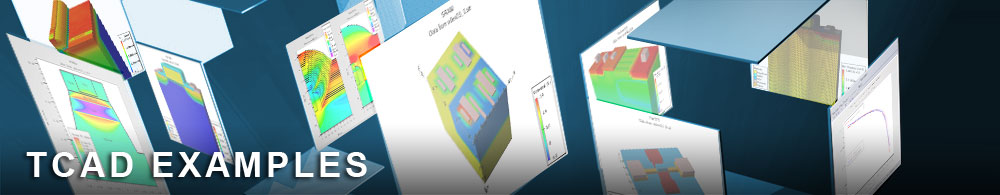Inductance Q Factor Optimization Using GA Algorithm

## vwfex10 : Inductance Q Factor Optimization Using GA Algorithm

Requires: VWF, QUEST

This example demonstrates how to optimize a curve using global optimizers (GA, SA, PT ..). The simplest way to do this is to optimize on a scalar target. DBinternal is used to compute the scalar value (i.e least square of relative error), and VWF always minimizes the target.

To open and run this example, select the Help Load example button in the VWF explorer. This will import all examples into your database. Once imported, the examples will be available under the directory called examples.